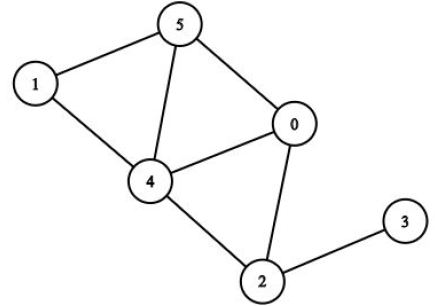# Least Constraining Value heuristic for graph coloring problem

The graph coloring problem involves assigning colors to vertices in a graph, such that no pair of adjacent vertices have the same color. I was given the problem to use least constraining value heuristic to determine the order in which the nodes would be colored.The image shows the graph that must be colored using the set of colors {a,b,c}, using least constraining value heuristic. From my understanding of the LCV heuristic, I am supposed to pick the vertex which eliminates the least amount of colors for its neighbors. We are also given the condition that for tie breaking, we use the smaller numbered node first, and the color 'a' first, 'b' second and 'c' third. Looking at each node, assigning color 'a' to vertex 3 only eliminates the possibility of vertex 2 being colored as 'a', while all other nodes cause 2 or more changes to their neighbors. Going forward, got the following order. 3a 0a 1a 2b 4c 5b But according to the key, the answer is supposed to be as follows. 0a 1a 2b 3a 4c 5b Could someone please explain what I'm doing wrong?